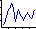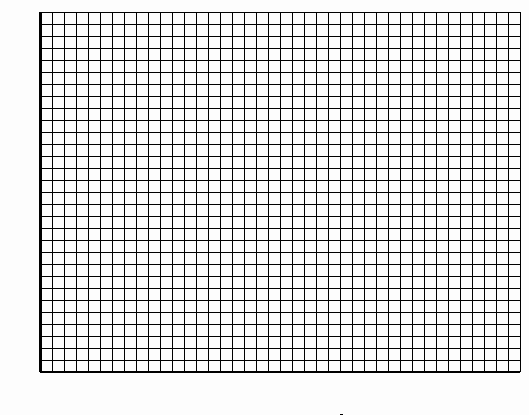PRINT THIS ANSWER SHEET TO FILL OUT AND TURN IN.

NAME_________________________________ CLASS____________________ DATE____________

## Density Activity Worksheet

This activity is designed to show the relationships among altitude or height above sea level, the density of the air, the temperature of the air, and the pressure of the air.

Data Table

 Altitude (meters) Density (kg/m3) 0 _____________ 100 _____________ _____________ _____________ _____________ _____________ _____________ _____________ _____________ _____________ _____________ _____________ _____________ _____________ _____________ _____________

Questions:1. Use the data that you recorded to produce a line graph. Use altitude as the independent variable and make a line of best fit.2. Use your graph to make a general statement on the relationship of density to altitude.

3. Why do you think this happens?

4. Convert the following altitudes from meters to feet.
a) 1000 meters =

b) 2500 meters =

c) 6500 meters =

5. After converting your altitudes to feet, you can calculate (or predict) the temperature at these altitudes by using the following equation: T = 59 - .00356 (h), where h = altitude (in feet). Your temperatures will be in degrees F. Calculate the temperature for each of the altitudes in Question 4.

a) temperature at 1000 meters =

b) temperature at 2500 meters =

c) temperature at 6500 meters =

6. Use AtmosModeler to check your answers. Be sure the "Units" menu says "Imperial" to compute in English units. Input each of the altitudes (in feet). Compare your answers for temperature to the AtmosModeler temperatures and explain how any differences may have occurred.

a)

b)

c)

7. The temperature may now be used to calculate the pressure of the air at each altitude. Using the equation p = 2116 (T + 459.7 / 518.6)5.256 where T = degrees F and p = lbs/in2, find the pressure that corresponds to each of your temperatures.

a) pressure =

b) pressure =

c) pressure =

8. How does the pressure change compared to a change in altitude?

9. Use a model or table to compare the change in altitude, density, temperature, and pressure at the same time. Include an explanation.